2.1 Tunneling — A Process Depending on Device Electrostatics

Since NBTI is triggered by the electric field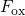across the dielectric, much importance is attached to the electrostatics within the device. Since source and drain are grounded during NBTI stress, the electrical potential remains almost constant along the interface so that the charge carriers face the same conditions for charge trapping over the entire channel area. As a consequence, the description of this process can be reduced to a one-dimensional problem (in the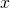-direction) where the electrostatics are only governed by the gate bias. The corresponding band diagram of a MOSFET with a p-doped silicon substrate biased in inversion is depicted in Fig. 2.1 (left). Due to the potential difference between substrate and gate, the band edges are strongly bent close to the interface so that the conduction band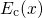forms a potential well. The electrons therein are confined to a small region close to the interface, which results in the build-up of discrete quasi-bound states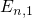,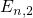,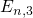,as shown in Fig. 2.1. The energetical separation between these states narrows towards higher energies and changes to a continuum of free states at the energy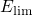. In the two other dimensions (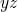-plane), the channel electrons behave as free particles and can therefore carry a current in these directions. The combination of quasi-bound and free states yields subbands as illustrated in Fig. 2.1 (right). Therefore, steps appear in the electron density of states (DOS)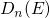, where each step belongs to one subband. The occupation probability of a state is given by Fermi-Dirac statistics, which apply as long as thermal equilibrium prevails. This is certainly the case for NBTI conditions, where the channel does not carry any appreciable current. Indeed, small and short channel current pulses, required in some measurement techniques to assess the NBTI degradation, lead to an overpopulation of high energy states. However, at the end of each pulse, the redistribution of the charge carriers back to equilibrium proceeds quickly and thus has not been noticed in experiments up to now. For this reason, electrons are assumed to obey the Fermi-Dirac statistics during NBTI conditions.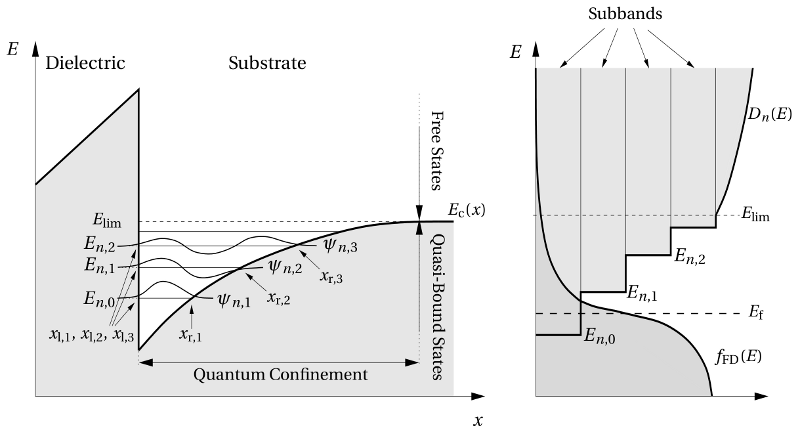Figure 2.1: Left: A schematic band diagram of the inversion layer. The wavefunctions of the quasi-bound states,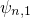,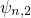,are spatially confined between the dielectric on the left-hand side and the conduction band on the right-hand side. The free states encounter no boundary on the right-hand side and are energetically located above the quasi-bound states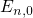,,,at the conduction band edge deep in the substrate (). Right: Schematic of the DOS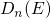and the Fermi-Dirac distribution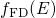. Each quasi-bound state associated with its own subband, resulting in a stepped DOS. The Fermi energy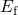places the Fermi-Dirac distribution relative to the DOS and determines the occupation of each state.

There exist several approximate methods to obtain the wavefunctions of bound states, however, each of them suffers from oversimplifications in certain regions. The first approach makes use of the Airy functions , which are solutions of the Schrödinger equation when the inversion layer is approximated by triangular potential well. Even though these functions satisfactorily reproduce the oscillatory behavior within the channel, they lack the exponential tails penetrating into the dielectric. This is due to the fact that the discontinuity at the interface is approximated by an infinitely high barrier. Another approach is provided by Gundlach’s method  that focuses on the part of the wavefunctions located within the dielectric. The channel electrons, however, are modeled as free particles in a constant potential and thus the effect of the electric field within the channel is not considered in this method. The third analytical approach relies on the Wentzel-Kramers-Brillouin (WKB) approximation  (see Appendix A.2), which stems from a semi-classical derivation. However, this approximation breaks down at the classical turning points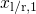,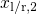,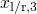where the energies of the quasi-bound states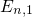,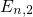,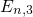,fall below the conduction band edge. This problem can be overcome using Langer’s method , which yields reasonable results — even in a region close to the classical turning points — and is thus frequently applied for the calculation of the tunneling probability. The most interesting part of the wavefunctions lies in the region left to the interface where the WKB approximation is often simplified assuming a trapezoidal, a triangular, or even a rectangular energy barrier (see Appendix A.3). The above deficiencies can be overcome by numerically solving the Schrödinger equation for the whole region including the substrate and the dielectric. This, for instance, is carried out in a Schrödinger-Poisson solver, which also considers the electrostatics within the device (see Chapter 3).

The injection of electrons into the dielectric is hindered in crystal and amorphous structures due to the absence of any quantum states within their bandgap. Atomic arrangements, where the symmetry of the regular structure is broken, are termed defects. During processing they unavoidably arise in large abundance and are distributed over the whole oxide. Furthermore, these defects have orbitals that can potentially introduce energy levels within the insulator bandgap and are thus capable of capturing and emitting substrate charge carriers. The band edges of the dielectric are large energy barriers for the charge carriers in the substrate. Since the band offset between the substrate and the dielectric has values of several electron volts, thermal activation over these barriers is negligible when there is only a small bias applied between source and drain. However, the wavefunctions of the charge carriers feature quickly decaying tails into the oxide. This implies a non-zero probability of encountering charge carriers within the dielectric, meaning that they penetrate into the dielectric and can be captured by defects. The rates of such transitions are given by Fermi’s golden rule.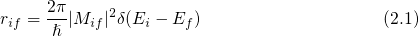The subscriptsand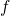denote the initial and the final state of the tunneling electron.is a matrix element, which is associated with the transition and can be calculated as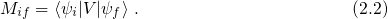The term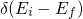in equation (2.1) guarantees energy conservation before and after the transition. This kind of process is commonly referred to as ‘elastic’, where this term only refers to the energy of the exchanged electron.

Tewksbury  provided an expression for matrix element assuming a constant oxide field within the dielectric and a constant potential within the substrate. In his derivation, the trap was approximated by a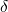-type potential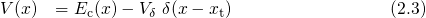with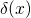being the Dirac delta function.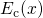and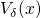are the conduction band edge in the oxide and the depth of the trap potential, respectively. The form of this potential implies that the integral in the matrix element only contributes directly at the center of the trap. Then the matrix element has the form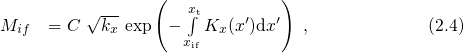where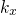and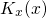are defined as the wavevectors within the substrate and the dielectric, respectively.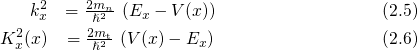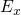denotes the energy of the electrons in the-direction and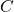is a normalization constant.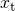stands for the spatial depth of a trap measured from the substrate-oxide interface and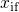is the position of the interface.represents the tunneling mass and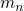is the electron mass in the conduction band. Note that the exponential term in equation (2.4) is the dominant factor in the matrix element. For slowly varying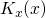, it decays with increasing trap depth — a behavior characteristic for all kinds of tunneling processes. The WKB integral in its exponent originates from the phase factor of the channel wavefunction, whose corresponding wavevector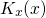is often approximated to be constant for simplicity . Due to the reduction to a one-dimensional problem, the-type trap potential covers the entire plane parallel to the interface. To correct for this shortcoming, Tewksbury introduced a capture cross section following the Freeman approach .

Lundstrom et al.   derived an expression of the matrix element assuming a step-potential for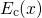and a three-dimensional-type trap potential .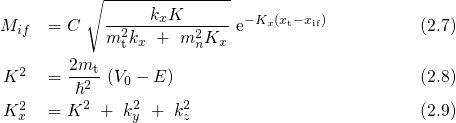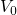stands for the height of the potential step. The wavevectors parallel to the interface are referred to as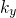and. Due to the constant potential energy within the dielectric, the WKB integrand is reduced to a simple multiplication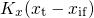. Keep in mind that the kinetic energy of the electrons perpendicular to the interface enters the exponential term in contrast to equation (2.4). The sum of this matrix element over all conduction band states assuming a free Fermi gas delivers a tunneling time constant of the form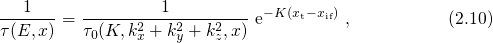where the prefactor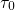is shown to weakly depend on the trap energy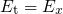and the trap depth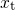. Note that wavevector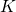, the equivalent quantity to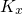in the one-dimensional case, enters the exponential term again.

The mechanism of pure elastic electron tunneling is often used as the standard explanation of charge trapping in MOSFETs. Over the time, several simplified expressions of tunneling rates have been published in numerous distinct charge trapping models  and will be touched on for completeness below. Christenson et al.   used Shockley-Read-Hall statistics in order to investigate the low frequency noise spectrum of a MOS transistor. His hole capture rates incorporate a tunneling probability through a rectangular potential barrier depending on the depth of a trap.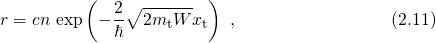where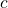and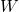stand for a capture coefficient and the barrier height, respectively. A further improvement has been achieved by Scharf , who accounted for the energy dependence of the tunneling charge carriers.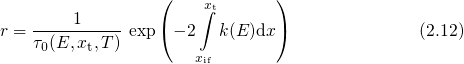Here, the integral extends from the semiconductor-oxide interfaceto trap depth. Note that the second factor on the right-hand side of equation (2.12) corresponds to a WKB factor and reflects the exponentially decaying tunneling probability with increasing trap depth. However, this factor has been phenomenologically introduced by Scharf but does not arise from a rigorous derivation. The approximation provided by Tewksbury is the most suited one for the application to MOSFETs since it accounts for the energy and depth dependence and uses a convenient one-dimensional formulation of tunneling.